# LSF correction factor for slanted-edge MTF measurements

April 1, 2015
April 14, 2018

A correction factor for the slanted-edge MTF (Edge SFR; E-SFR) calculations in SFR, SFRplus, eSFR ISO, SFRreg, and Checkerboard was added to Imatest 4.1.2 (March 2015). This correction factor is included in the ISO 12233:2014 standard, but is not in the older ISO 12233:2000 standard. Because it corrects for an MTF loss caused by the numerical calculation of the Line Spread Function (LSF) from the Edge Spread Function (ESF), we call it the LSF correction factor.

The LSF correction factor primarily affects very high spatial frequencies beyond most of the energy for typical high quality cameras. But it does make a difference for practical measurements: MTF50 for a typical high quality camera (shown below) is increased by about 1.5%.

The correction factor was turned off by default in Imatest versions 4.1.n. It is turned on by default in versions 4.2+. It can be set (to override the default) by pressing Settings, Options III in the Imatest main window as shown below, then checking the box for the correction. We strongly recommend turning on the LSF correction factor, i.e., the box should be checked.

### The equations

Here is the Edge SFR (MTF) equation from ISO 12233:2000, Annex C:And here is the corresponding equation from ISO 12233:2014, Annex D. Note that E-SFR(k) is the symbol for edge-based SFR, not a subtraction: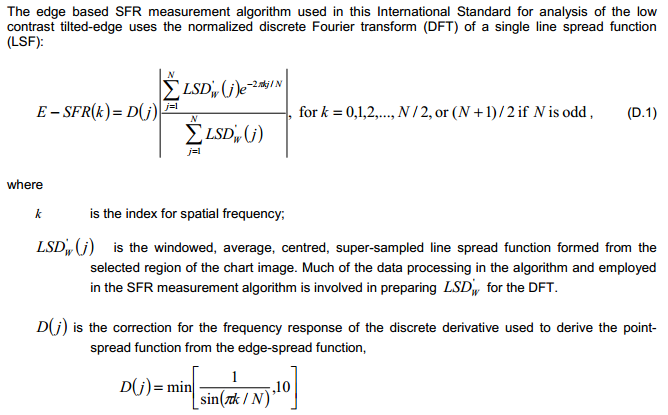The difference between the two equations is the LSF correction factor D(j) for the numerical differentiation used to calculate the Line Spread (not Point Spread*) Function from the Edge Spread Function. Note that D(j) is incorrect in the ISO 12233:2014 standard. It should read,

 $$D(j) = \text{min}\left[\frac{\pi k / N}{\sin\left(\pi k / N\right)}, 10\right]$$ *If you thought ISO standards were written by gods on Mount Olympus (the Greek version), the many misprints should set you straight.

 Numerical differentiation is a linear process with a transfer function that differs from ideal differentiation. The ISO 12233:2014 formula (D.8) for calculating the Line Spread Function LSF(x) from the 4x-oversampled Edge Spread Function ESF(x) is, $$LSF_W(j) = W(j)\frac{ESF(j+1) – ESF(j-1)}{2}$$ where W(j) is a windowing function not relevant to this analysis. Note that the spacing between points in this calculation is 2 (4x-oversampled) samples = 0.5 pixels. This numerical difference formula may be rewritten, $$LSF(x) = \frac{ESF(x + \Delta x/2) – ESF(x – \Delta x/2)}{\Delta x}$$     where Δx  = 0.5 pixels. The Fourier transform (FT) for a time (or spatial) shift is given in Wikipedia. $$FT(g(x-a)) = e^{-ia\omega} FT(g(x))$$ For numerical differentiation, the Fourier transform is $$\displaystyle FT_{numerical} = \frac{FT(g(x+a/2)-g(x-a/2))}{a} = \frac{e^{ia\omega/2}-e^{-ia\omega/2}}{a}FT(g(x))$$ Noting that  $$\sin(x) = \frac{e^{ix}-e^{-ix}}{2i}$$  (see Wikipedia), $$FT_{numerical} = \frac{2\sin(a\omega /2)}{a}FT(g(x))$$ For pure differentiation, the Fourier transform is $$FT_{pure} = FT\left(\frac{d g(x)}{dx}\right) = \omega FT(g(x))$$ The correction factor is therefore $$D(\omega) = \left|\frac{FT_{pure}}{FT_{numerical}}\right| = \frac{a\omega /2}{\sin(a\omega /2)}$$ Since Δx = a = 0.5 pixels for the 4x-oversampled signal, and frequency f = ω/(2π) has units of cycles/pixel, $$D(f) = \left|\frac{FT_{pure}}{FT_{numerical}}\right| = \frac{\pi f/2}{\sin(\pi f/2)}$$ At the Nyquist frequency, fNyq = 0.5 Cycles/Pixel, $$D = \frac{\pi /4}{\sin(\pi /4)} = 1.1107$$. At 2*Nyquist = 1 C/P, D = π/2 = 1.5708.  To obtain D(j), substitute πk/N for aω/2.  min[…, 10] keeps D(j) from becoming excessive where (πk/N ) >> sin(πk/N ).

### Applying the correction factor

To apply (or disable) the LSF correction factor, click Settings (in the Imatest main window), Options III. Check or uncheck the Use LSF… checkbox as appropriate. The checkbox sets derivCorr in the [imatest] section of imatest-v2.ini to 0 (correction off) or 1 (correction on).Options III window for applying or removing LSF correction factor

For most applications we recommend checking the box– turning on the correction factor. However, for industrial applications that use fixed pass/fail thresholds, consistency may be more important than accuracy. To prevent changes in yield and/or avoid modifications to the pass/fail specification, the box should remain unchecked and devCorr = 0 should be added to the [imatest] section of the ini file.

###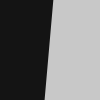Verification

To observe the effects of the LSF correction factor, we use an idealized edge, tilted 5 degrees, shown on the right. You can click on it to download it for your own testing.

The ideal edge increases uniformly from 0 to 1 over a distance of τ = 1 pixel.

 The MTF of an ideal uniformly-increasing edge of width τ is the Fourier transform FT of its derivative, which is $$f(t) = \frac{u\left((t+\tau) / 2\right) – u\left((t-\tau) / 2\right)}{\tau}$$     for unit step function u. $$FT(f) = \frac{\sin(\pi \tau f)}{\pi \tau f}$$ If τ is the sampling rate (the same as Δx or a in the green box, above), $$\textrm{Nyquist frequency} = f_{\textrm{Nyq}} = \frac{1}{2\tau}$$ The expected value of MTF at the Nyquist frequency (0.5 cycles/pixel) is $$\displaystyle FT(f_{\textrm{Nyq}}) = \frac{\sin(\pi\tau f_{\textrm{Nyq}})}{\pi\tau f_{\textrm{Nyq}}} = \frac{\sin(\pi /2)}{\pi /2} = \frac{2}{\pi} = 0.6366$$

Here are the results without and with the LSF correction factor. Note that gamma has been set to 1 because the idealized image is not gamma-encoded. The results with LSF correction are much closer to the expected value of 2/π = 0.6366. The difference is likely due to digital sampling and the numerical binning/oversampling process: the average oversampled edge shown in the figures below is slightly rounded. Also, the edge rotation correction that is not applied to edges slanted by less than 8 degrees. The edge rotation is not included in the ISO standard and so is not applied to edges that fall under the ISO standard algorithm.Ideal edge, uncorrected MTF. MTF@Nyquist = 0.56.Ideal edge, LSF-corrected MTF. MTF@Nyquist = 0.624.

 The figure on the right, generated by MTF Compare (a postprocessor to MTF calculation programs for comparing MTF calculations), compares the uncorrected MTF of the ideal edge (blue) with the corrected MTF (burgundy). The black line is the uncorrected/corrected transfer function = 1/(correction factor D). It has the expected values of 0.9 (1/1.1107) at the Nyquist frequency (f = 0.5 C/P) and 2/π = 0.6366 at 2*Nyquist (1 C/P).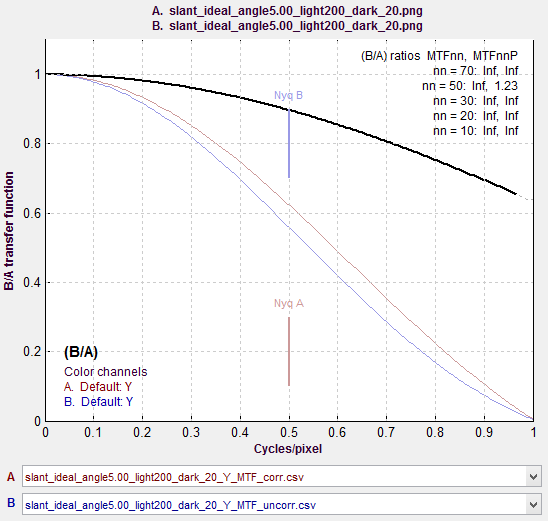MTF Compare Uncorrected vs. Corrected

##### Effects of gamma

Most color space files are gamma-encoded with gamma around 0.5, which is the default for Imatest slanted-edge SFR calculations. (Actual gamma varies considerably, and may be complicated by a tonal response curve on top of the gamma curve.)  However, the ideal pulse is not gamma-encoded, so gamma = 1 is the appropriate setting. If gamma is set to 0.5 (the default), an inappropriate linearization will be applied to the edge, resulting in erroneous results: the MTF at 2*Nyquist is not equal to 0, as it should be.Ideal edge, uncorrected MTF, calculated
(incorrectly) with gamma = 0.5

The idealized image has much more energy above the Nyquist frequency than typical high quality images. Here is an example for a high quality camera showing the effects of the LSF correction factor on MTF50— the most commonly-used summary metric.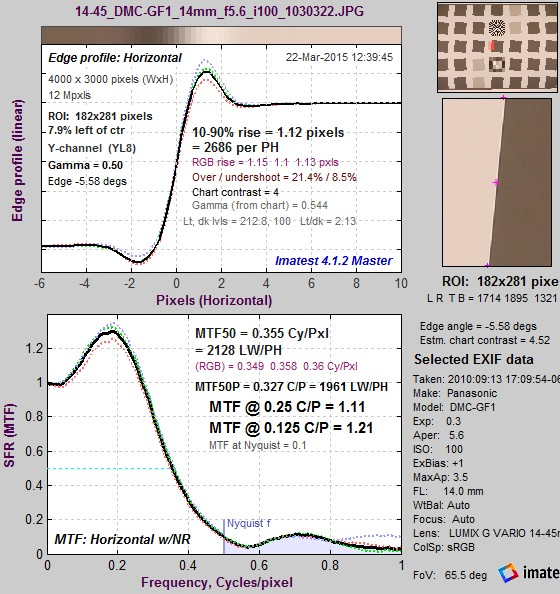Typical edge, uncorrected MTF. MTF50 = 0.355 Cycles/Pixel.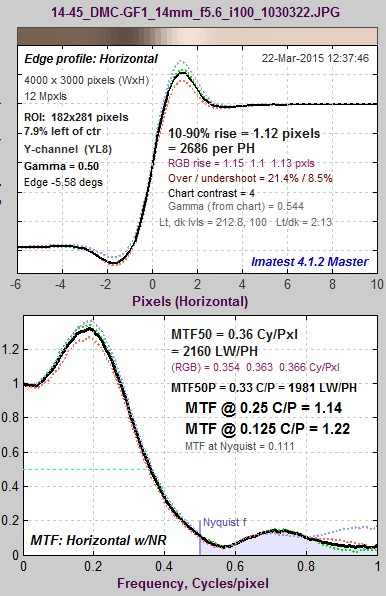Typical edge, LSF-corrected MTF. MTF50 = 0.360 C/P:  1.41% higher.

The difference may not be significant for many applications.

### Consistency with older Imatest versions

The new correction factor is available in Imatest 4.1.2+, where it is turned off by default. The correction factor is turned on by default in Imatest 4.2+ releases.

You can override the default, i.e., turn the correction factor on or off, by clicking Settings (in the Imatest main window), Options III, and checking or unchecking the Use LSF… checkbox as appropriate. Once the setting is saved, it will be retained across all future versions unless changed by the user. The default calculation be selected unless you manually change it in Options III window.

We strongly recommend turning on the LSF correction factor, i.e., the box should be checked.

For industrial testing with pass/fail thresholds set up with the old calculation, we recommend either: